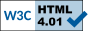## CMSC 39600: PCPs, codes and inapproximability - Autumn 2007

### Probabilitically Checkable Proofs, error correcting codes and inapproximability results

Time: Tue/Thu 12:00-1:20pm
Location: TTI-C Conference Room (second floor of University Press Building)
Instructor : (<firstname> AT tti-c DOT org)

#### Problem Sets

1. Problem Set 1 [ pdf | ps ] (Due: Nov 8, 2007)
2. Problem Set 2 [ pdf | ps ] (Due: Dec 6, 2007)

#### Lectures

1. Lecture 1 (Sep 25): The PCP Theorem -- Introduction and two views.
PCP Theorem -- two views (a) hardness of approximation and (b) proof checking. Equivalence of PCP Theorem in the two views.
(Notes: [pdf]; Scribe Notes (by Josh Grochow): [ pdf | ps ]

2. Lecture 2 (Sep 27): PCPs -- definition and Inapproximability of Clique.
Definition of PCP Classes, PCP Theorem and its various strengthenings, inapproximability of clique.
(Notes: [pdf]; Scribe Notes (By Karthik Sridharan): [ pdf | ps ])

3. Lecture 3 (Oct 2): Linearity Testing.
Coding Theory - Prelims, Walsh-Hadamard code, linearity testing, Fourier analysis
(Notes: [pdf]; Scribe Notes (By Josh Grochow): [ pdf | ps ])

4. Lecture 4 (Oct 4): NP \subseteq PCP(poly, O(1))
Exponential sized PCPs for NP, PCPs of proximity.
(Notes: [pdf]; Scribe Notes (By Andy Cotter): [ pdf | ps ]

5. Lecture 5 (Oct 9): Proof Composition
PCPs of Proximity, Robust PCPs, Proof Composition, Introduction to Dinur's Proof of the PCP Theorem.
(Notes: [pdf]; Scribe Notes (By Bill Fefferman): [ pdf | ps ])

6. Lecture 6 (Oct 11): Dinur's proof of the PCP Theorem (Part 1)
Overview of Dinur's proof; Expander - Preliminaries; Phase I: Preprocessing (degree reduction).
(Notes: [pdf]; Scribe Notes: see below)

7. Lecture 7 (Oct 16): Dinur's proof of the PCP Theorem (Part 2)
Phase I: Preprocessing (degree reduction, expanderization), Phase II: Graph Powering.
(Scribe Notes: see below)

8. Lecture 8 (Oct 18): Dinur's proof of the PCP Theorem (Part 3)
Phase II: Graph Powering, Phase III: Proof Composition (alphabet reduction).
(Scribe Notes (for Lec 6,7 & 8): [ pdf | ps ])

Oct 23: No Lecture (FOCS 2007); Problem Set 1 Out

9. Lecture 9 (Oct 25): Label Cover Problem
Label Cover problem, 2 prover 1 round (2P1R) games, introduction to parallel repetition theorem.
(Scribe Notes (by Parinya Chalermsook): )

10. Lecture 10 (Oct 30): 3-query PCPs for NP
Hardness of approximating MAX-3LIN2, 3 query PCPs for NP, Long code and testing.
(Notes: [pdf]; Scribe Notes (by Karthik Sridharan: )

11. Lecture 11 (Nov 1): Proof of Parallel Repetition Theorem (Part 1)
Parallel Repetition Theorem - part I, some preliminary lemmas
(Notes: pdf; Scribe Notes (by Andy Cotter): )

12. Lecture 12 (Nov 6): Proof of Parallel Repetition Theorem (part 2)
(Notes: see notes for Lec 11; Scribe Notes (by Allie Shapiro): )

13. Lecture 13 (Nov 8): Low Degree Testing (Part 1)
Low degree testing, Plane-point test, Raz-Safra soundness analysis
(Notes: pdf; Scribe Notes (by Parinya Chalermsook):)

14. Lecture 14 (Nov 13): Low Degree Testing (Part 2)
Plane-point test, Raz-Safra soundness analysis
(Notes: pdf; Scribe Notes (by Bill Fefferman):)

15. Lecture 15 (Nov 15): (Original) Proof of the PCP Theorem using algebra (Part 1)
(Notes: pdf; Scribe Notes (by Allie Shapiro):)

16. Lecture 16 (Nov 15): (Original) Proof of the PCP Theorem using algebra (Part 2)
(Notes: pdf; Scribe Notes (by Josh Grochow):)

Nov 20: No lecture; Problem Set 2 Out

Tentative Topics for future lectures

17. Lecture 17 (Nov 27): (Guest Lecturer: Julia Chuzhoy) Unique Games Conjecture; UGC-hardness

Problem Set 2 Due (Dec 6)

Style files for scribes: sample.tex and preamble.tex.

#### Requirements

Students taking the course for credit will be expected to:

• Attend lectures
• Scribe notes for two lectures in LATEX (style files: sample.tex and preamble.tex).
• Do problem sets (2 problems sets at end of Oct and Nov)

This page has been accessed at leasttimes since September 15, 2007.
 Prahladh Harsha